# Quadratic equation - math problems

Mathematical word problems allow you to practice your mathematics knowledge in everyday life tasks. Word problems train to understand, translate into the mathematical language (e.g., equations), solve it, and check the accuracy and solution discussion.
Choose a topic you want to calculate and improve in.

### From our database of math word problems and examples we offer:

• In theIn the national park, the ratio of the wooded area to grassland is 4: 1. The total area is 385km2. What area is wooded?3 3/4 + 2 3/5 + 5 1/2 Show your solution.
• Wheel gearA drive wheel of radius 2 is connected to a drive wheel of radius 1 by a pulley of length 17. What is the distance between the wheel axles?
• Circle and squareAn ABCD square with a side length of 100 mm is given. Calculate the radius of the circle that passes through the vertices B, C and the center of the side AD.
• Ali boughtAli bought 5/6 litre of milk. He drank 1/2 litre and his brother drank 1/6 litre. How much litre of milk left?
• Peter 2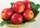Peter wanted to have 120 kilogram of fruits.  He picked 10 bags .  each bags contains 9 1/2 kilograms. How many kilograms he need more?
• Celsius 25 degrees Celsius at midday dropped 12°Celsius degree by evening. What is the temperature?
• ContainerThe container-shaped box with internal dimensions of 3.9 m, 3.25 m and 2.6 m was completely filled with goods in the same cubic boxes. How long edge could this box have?The ladder touch on a wall at a height of 7.5 m. The angle of the inclination of the ladder is 76°. How far is the lower end of the ladder from the wall?
• The heights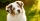The heights of some dogs are shown in the table. (1 cm = 10 mm) Dog Height Sam 280 mm Charlie 56 cm Gus 81.3 cm Buster 635 mm Max 38 cm Duke 61 cm Which list shows the heights of the dogs from tallest to shortest? A. Max, Charlie, Duke, Gus, Sam,
• Prism - eq triangleCalculate the volume and surface of the prism with the base of an equilateral triangle with side a = 4cm and the body height is 6cm.
• Edges of the cuboid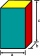Find the length of the edges of the cuboid, which has the following dimensions: width is 0.4 m; the height is 5.8 dm and the block can hold 81.2 liters of fluid.
• CuboidFind the dimensions of cuboid a, b, c; if diagonal d=9 dm has an angle with edge α=55° and has an angle with edge b β=58°
• Pagans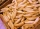Jano and Michael ate pagans. Jano ate three more than Michael. The product of their counts (numbers) is 180. How many pagans did each of them eat?
• English and math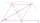1/5 of a class study English, and 3/5 study mathematics. If the class consist of 80 students, how many students study English and mathematics in the class?
• Clay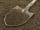How many cubic centimeters of clay is in a pit of dimensions 4 m x 3 m x 3 m?
• Open intervals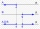Open intervals A = (x-2; 2x-1) and B = (3x-4; 4) are given. Find the largest real number for which A ⊂ B applies.